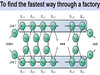dynamic programming

DP

《Dynamic Programming》

• 动态规划（Dynamic programming，简称DP）很多人都觉得是比较难以理解和掌握的一种算法，为了应付面试更多的时候程序员会选择直接死记硬背斐波那楔数列或者背包问题的源码，其实只要认真学习、彻底理解，动态规划并...python工程师 python爬虫 python python教程 python安装
• 文章目录1.序2.动态规划的基本概念[^1]3.动态规划算法的基本思想[^2]4....这篇文章主要介绍动态规划算法的基本思想、使用动态规划算法求解问题的基本步骤、动态规划算法的两个基本要素以及一些经典的动态规划问题。...
文章目录1.序2.动态规划的基本概念[^1]3.动态规划算法的基本思想[^2]4.动态规划的求解步骤[^2]5.动态规划算法的基本要素[^2]5.1 最优子结构5.2 重叠子问题6.一些经典的动态规划问题
1.序
近期笔者会写一些博客，与大家共同讨论一些经典的算法思想。这篇文章主要介绍动态规划算法的基本思想、使用动态规划算法求解问题的基本步骤、动态规划算法的两个基本要素以及一些经典的动态规划问题。
2.动态规划的基本概念[^1]
在学习动态规划之前，先思考这样一个问题：什么是动态规划？为什么叫动态规划？
当读者在试图探索这个问题的时候，不可避免的要了解动态规划的产生背景。动态规划是由 Dynamic Programming 翻译过来的。动态规划的概念是由美国数学家R.E.Bellman等人提出的，应用于工程领域。
动态规划是是求解多阶段决策过程(decision process)的最优化问题一种方法。
所谓多阶段决策过程是指这样一类决策过程：它可以把一一个复杂问题按时间(或空间)分成若干个阶段，每个阶段都需要作出决策，
以便得到过程的最优结局。由于在每阶段采取的决策是与时间有关的而且前一阶段采取的决策如何，不但与该阶段的经济效果有关，
还影响以后各阶段的经济效果，可见这类多阶段决策问题是一个动态的问题，因此，处理的方法称为动态规划方法。然而，动态
规划也可以处理一些本来与时间没有关系的静态模型，这只要在静态模型中人为地引入“时间”因素，分成时段，就可以把它看作
是多阶段的动态模型，用动态规划方法去处理。

简言之，多阶段决策过程是指这样的一类特殊的活动过程：问题可以按时间顺序分解成若干相互联系的阶段，在每一个阶段都要做出决策，全部过程的决策是一个决策序列。
下面举例说明什么是多阶段决策问题。
例1（最短路线问题）在线路网络图1中，从A至E有一批货物需要调运。图上所标数字为各节点之间的运输距离，为使总运费最少，必须找出一条由A至E总里程最短的路线。图1
为了找到由A至E的最短线路，可以将该问题分成A—B—C—D—E 4个阶段，在每个阶段都需要作出决策，即在A点需决策下一步到B1还是到B2或B3；同样，若到达第二阶段某个状态，比如B1 ，需决定走向C1还是C2 ；依次类推，可以看出：各个阶段的决策不同，由A至E的路线就不同，当从某个阶段的某个状态出发作出一个决策，则这个决策不仅影响到下一个阶段的距离，而且直接影响后面各阶段的行进线路。所以这类问题要求在各个阶段选择一个恰当的决策，使这些决策序列所决定的一条路线对应的总路程最短。
3.动态规划算法的基本思想[^2]
动态规划算法与分治法类似，其基本思想也是将待求解问题分解成若干个子问题。图2
但是经分解得到的子问题往往不是互相独立的。不同子问题的数目常常只有多项式量级。在用分治法求解时，有些子问题被重复计算了许多次。
如果能够保存已解决的子问题的答案，而在需要时再找出已求得的答案，就可以避免大量重复计算，从而得到多项式时间算法。图3
4.动态规划的求解步骤[^2]
a. 找出最优解的性质，并刻划其结构特征。
b. 递归地定义最优值。
c. 以自底向上的方式计算出最优值。
d. 根据计算最优值时得到的信息，构造最优解
5.动态规划算法的基本要素[^2]
5.1 最优子结构

矩阵连乘计算次序问题的最优解包含着其子问题的最优解。这种性质称为最优子结构性质。
在分析问题的最优子结构性质时，所用的方法具有普遍性：首先假设由问题的最优解导出的子问题的解不是最优的，然后再设法说明在这个假设下可构造出比原问题最优解更好的解，从而导致矛盾。
利用问题的最优子结构性质，以自底向上的方式递归地从子问题的最优解逐步构造出整个问题的最优解。最优子结构是问题能用动态规划算法求解的前提。

注意：同一个问题可以有多种方式刻划它的最优子结构，有些表示方法的求解速度更快（空间占用小，问题的维度低）
5.2 重叠子问题

递归算法求解问题时，每次产生的子问题并不总是新问题，有些子问题被反复计算多次。这种性质称为子问题的重叠性质。
动态规划算法，对每一个子问题只解一次，而后将其解保存在一个表格中，当再次需要解此子问题时，只是简单地用常数时间查看一下结果。
通常不同的子问题个数随问题的大小呈多项式增长。因此用动态规划算法只需要多项式时间，从而获得较高的解题效率。图4

6.一些经典的动态规划问题
这部分待补充~
笔者要充电了~
参考文献
 引用自百度文库https://wenku.baidu.com/view/c0f9fb156c175f0e7cd1377d.html
引用自老师的课件


展开全文动态规划 动态规划基本思想
• 相对于我来说，算法里面遇到的问题里面感觉最难的也就是动态规划（Dynamic Programming）算法了，于是花了好长时间，查找了相关的文献和资料准备彻底的理解动态规划（Dynamic Programming）算法。一是帮助自己总结...
前言

最近在牛客网上做了几套公司的真题，发现有关动态规划（Dynamic Programming）算法的题目很多。相对于我来说，算法里面遇到的问题里面感觉最难的也就是动态规划（Dynamic Programming）算法了，于是花了好长时间，查找了相关的文献和资料准备彻底的理解动态规划（Dynamic Programming）算法。一是帮助自己总结知识点，二是也能够帮助他人更好的理解这个算法。后面的参考文献只是我看到的文献的一部分。

动态规划算法的核心

理解一个算法就要理解一个算法的核心，动态规划算法的核心是下面的一张图片和一个小故事。A * "1+1+1+1+1+1+1+1 =？" *

A : "上面等式的值是多少"
B : *计算* "8!"

A *在上面等式的左边写上 "1+" *
A : "此时等式的值为多少"
B : *quickly* "9!"
A : "你怎么这么快就知道答案了"
A : "只要在8的基础上加1就行了"
A : "所以你不用重新计算因为你记住了第一个等式的值为8!动态规划算法也可以说是 '记住求过的解来节省时间'"

由上面的图片和小故事可以知道动态规划算法的核心就是记住已经解决过的子问题的解。

动态规划算法的两种形式

上面已经知道动态规划算法的核心是记住已经求过的解，记住求解的方式有两种：①自顶向下的备忘录法  ②自底向上。
为了说明动态规划的这两种方法，举一个最简单的例子：求斐波拉契数列Fibonacci 。先看一下这个问题：

Fibonacci (n) = 1;   n = 0

Fibonacci (n) = 1;   n = 1

Fibonacci (n) = Fibonacci(n-1) + Fibonacci(n-2)

以前学c语言的时候写过这个算法使用递归十分的简单。先使用递归版本来实现这个算法：

public int fib(int n)
{
if(n<=0)
return 0;
if(n==1)
return 1;
return fib( n-1)+fib(n-2);
}
//输入6
//输出：8


先来分析一下递归算法的执行流程，假如输入6，那么执行的递归树如下：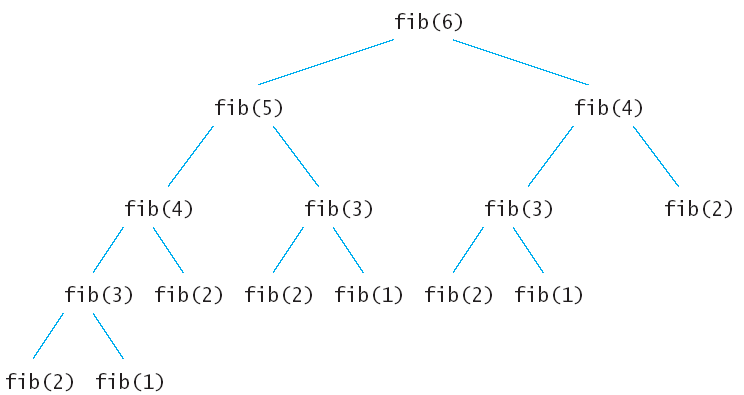上面的递归树中的每一个子节点都会执行一次，很多重复的节点被执行，fib(2)被重复执行了5次。由于调用每一个函数的时候都要保留上下文，所以空间上开销也不小。这么多的子节点被重复执行，如果在执行的时候把执行过的子节点保存起来，后面要用到的时候直接查表调用的话可以节约大量的时间。下面就看看动态规划的两种方法怎样来解决斐波拉契数列Fibonacci 数列问题。

①自顶向下的备忘录法

public static int Fibonacci(int n)
{
if(n<=0)
return n;
int []Memo=new int[n+1];
for(int i=0;i<=n;i++)
Memo[i]=-1;
return fib(n, Memo);
}
public static int fib(int n,int []Memo)
{

if(Memo[n]!=-1)
return Memo[n];
//如果已经求出了fib（n）的值直接返回，否则将求出的值保存在Memo备忘录中。
if(n<=2)
Memo[n]=1;

else Memo[n]=fib( n-1,Memo)+fib(n-2,Memo);

return Memo[n];
}

备忘录法也是比较好理解的，创建了一个n+1大小的数组来保存求出的斐波拉契数列中的每一个值，在递归的时候如果发现前面fib（n）的值计算出来了就不再计算，如果未计算出来，则计算出来后保存在Memo数组中，下次在调用fib（n）的时候就不会重新递归了。比如上面的递归树中在计算fib（6）的时候先计算fib（5），调用fib（5）算出了fib（4）后，fib（6）再调用fib（4）就不会在递归fib（4）的子树了，因为fib（4）的值已经保存在Memo中。

②自底向上的动态规划

备忘录法还是利用了递归，上面算法不管怎样，计算fib（6）的时候最后还是要计算出fib（1），fib（2），fib（3）……,那么何不先计算出fib（1），fib（2），fib（3）……,呢？这也就是动态规划的核心，先计算子问题，再由子问题计算父问题。

public static int fib(int n)
{
if(n<=0)
return n;
int []Memo=new int[n+1];
Memo=0;
Memo=1;
for(int i=2;i<=n;i++)
{
Memo[i]=Memo[i-1]+Memo[i-2];
}
return Memo[n];
}

自底向上方法也是利用数组保存了先计算的值，为后面的调用服务。观察参与循环的只有 i，i-1 , i-2三项，因此该方法的空间可以进一步的压缩如下。

public static int fib(int n)
{
if(n<=1)
return n;

int Memo_i_2=0;
int Memo_i_1=1;
int Memo_i=1;
for(int i=2;i<=n;i++)
{
Memo_i=Memo_i_2+Memo_i_1;
Memo_i_2=Memo_i_1;
Memo_i_1=Memo_i;
}
return Memo_i;
}

一般来说由于备忘录方式的动态规划方法使用了递归，递归的时候会产生额外的开销，使用自底向上的动态规划方法要比备忘录方法好。
你以为看懂了上面的例子就懂得了动态规划吗？那就too young too simple了。动态规划远远不止如此简单，下面先给出一个例子看看能否独立完成。然后再对动态规划的其他特性进行分析。

动态规划小试牛刀

例题：钢条切割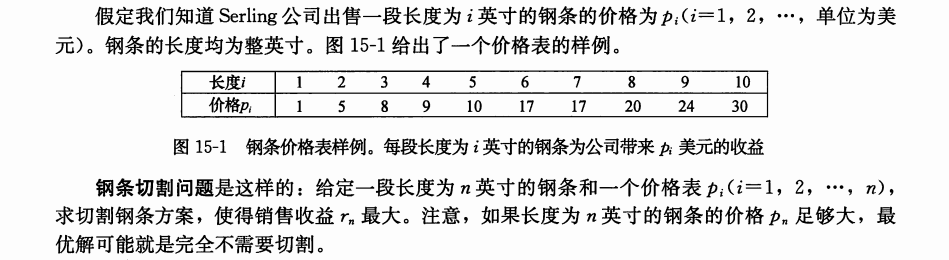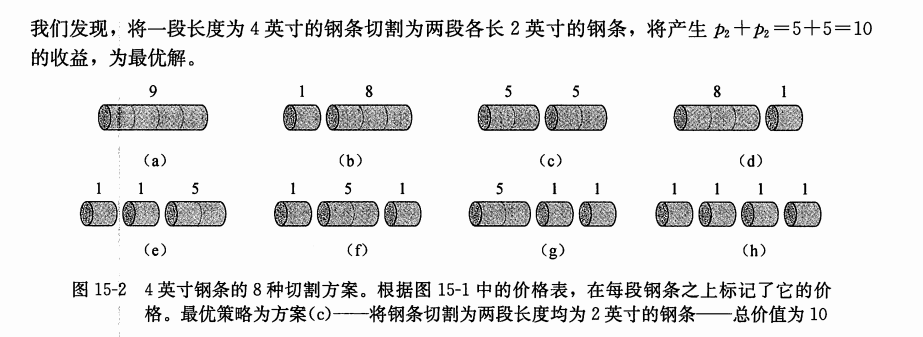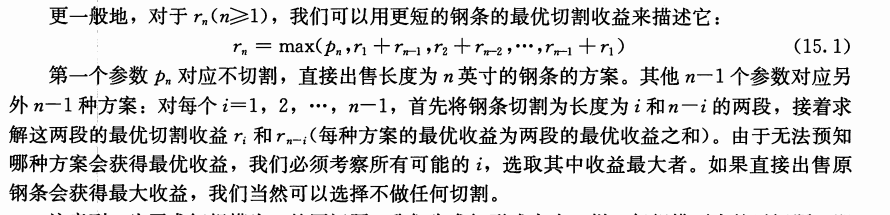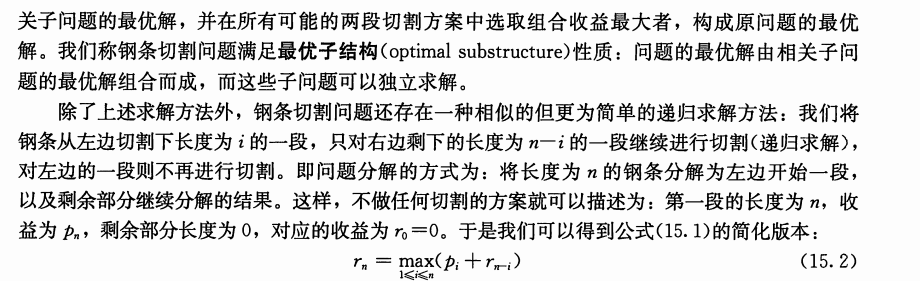上面的例题来自于算法导论
关于题目的讲解就直接截图算法导论书上了这里就不展开讲。现在使用一下前面讲到三种方法来来实现一下。 ①递归版本

public static int cut(int []p,int n)
{
if(n==0)
return 0;
int q=Integer.MIN_VALUE;
for(int i=1;i<=n;i++)
{
q=Math.max(q, p[i-1]+cut(p, n-i));
}
return q;
}

递归很好理解，如果不懂可以看上面的讲解，递归的思路其实和回溯法是一样的，遍历所有解空间但这里和上面斐波拉契数列的不同之处在于，在每一层上都进行了一次最优解的选择，q=Math.max(q, p[i-1]+cut(p, n-i));这个段语句就是最优解选择，这里上一层的最优解与下一层的最优解相关。

②备忘录版本

public static int cutMemo(int []p)
{
int []r=new int[p.length+1];
for(int i=0;i<=p.length;i++)
r[i]=-1;
return cut(p, p.length, r);
}
public static int cut(int []p,int n,int []r)
{
int q=-1;
if(r[n]>=0)
return r[n];
if(n==0)
q=0;
else {
for(int i=1;i<=n;i++)
q=Math.max(q, cut(p, n-i,r)+p[i-1]);
}
r[n]=q;

return q;
}

有了上面求斐波拉契数列的基础，理解备忘录方法也就不难了。备忘录方法无非是在递归的时候记录下已经调用过的子函数的值。这道钢条切割问题的经典之处在于自底向上的动态规划问题的处理，理解了这个也就理解了动态规划的精髓。

③自底向上的动态规划

public static int buttom_up_cut(int []p)
{
int []r=new int[p.length+1];
for(int i=1;i<=p.length;i++)
{
int q=-1;
//①
for(int j=1;j<=i;j++)
q=Math.max(q, p[j-1]+r[i-j]);
r[i]=q;
}
return r[p.length];
}

自底向上的动态规划问题中最重要的是理解注释①处的循环，这里外面的循环是求r,r……，里面的循环是求出r,r……的最优解，也就是说r[i]中保存的是钢条长度为i时划分的最优解，这里面涉及到了最优子结构问题，也就是一个问题取最优解的时候，它的子问题也一定要取得最优解。下面是长度为4的钢条划分的结构图。我就偷懒截了个图。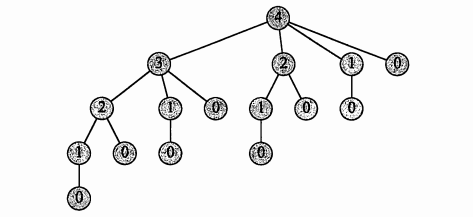动态规划原理

虽然已经用动态规划方法解决了上面两个问题，但是大家可能还跟我一样并不知道什么时候要用到动态规划。总结一下上面的斐波拉契数列和钢条切割问题，发现两个问题都涉及到了重叠子问题，和最优子结构。

①最优子结构

用动态规划求解最优化问题的第一步就是刻画最优解的结构，如果一个问题的解结构包含其子问题的最优解，就称此问题具有最优子结构性质。因此，某个问题是否适合应用动态规划算法，它是否具有最优子结构性质是一个很好的线索。使用动态规划算法时，用子问题的最优解来构造原问题的最优解。因此必须考查最优解中用到的所有子问题。

②重叠子问题

在斐波拉契数列和钢条切割结构图中，可以看到大量的重叠子问题，比如说在求fib（6）的时候，fib（2）被调用了5次，在求cut（4）的时候cut（0）被调用了4次。如果使用递归算法的时候会反复的求解相同的子问题，不停的调用函数，而不是生成新的子问题。如果递归算法反复求解相同的子问题，就称为具有重叠子问题（overlapping subproblems）性质。在动态规划算法中使用数组来保存子问题的解，这样子问题多次求解的时候可以直接查表不用调用函数递归。

动态规划的经典模型

线性模型

线性模型的是动态规划中最常用的模型，上文讲到的钢条切割问题就是经典的线性模型，这里的线性指的是状态的排布是呈线性的。【例题1】是一个经典的面试题，我们将它作为线性模型的敲门砖。

【例题1】在一个夜黑风高的晚上，有n（n <= 50）个小朋友在桥的这边，现在他们需要过桥，但是由于桥很窄，每次只允许不大于两人通过，他们只有一个手电筒，所以每次过桥的两个人需要把手电筒带回来，i号小朋友过桥的时间为T[i]，两个人过桥的总时间为二者中时间长者。问所有小朋友过桥的总时间最短是多少。每次过桥的时候最多两个人，如果桥这边还有人，那么还得回来一个人（送手电筒），也就是说N个人过桥的次数为2*N-3（倒推，当桥这边只剩两个人时只需要一次，三个人的情况为来回一次后加上两个人的情况…）。有一个人需要来回跑，将手电筒送回来（也许不是同一个人，realy？！）这个回来的时间是没办法省去的，并且回来的次数也是确定的，为N-2，如果是我，我会选择让跑的最快的人来干这件事情，但是我错了…如果总是跑得最快的人跑回来的话，那么他在每次别人过桥的时候一定得跟过去，于是就变成就是很简单的问题了，花费的总时间：

T = minPTime * (N-2) + (totalSum-minPTime)

来看一组数据 四个人过桥花费的时间分别为 1 2 5 10，按照上面的公式答案是19，但是实际答案应该是17。

具体步骤是这样的：

第一步：1和2过去，花费时间2，然后1回来（花费时间1）；

第二歩：3和4过去，花费时间10，然后2回来（花费时间2）；

第三部：1和2过去，花费时间2，总耗时17。

所以之前的贪心想法是不对的。我们先将所有人按花费时间递增进行排序，假设前i个人过河花费的最少时间为opt[i]，那么考虑前i-1个人过河的情况，即河这边还有1个人，河那边有i-1个人，并且这时候手电筒肯定在对岸，所以opt[i] = opt[i-1] + a + a[i]        (让花费时间最少的人把手电筒送过来，然后和第i个人一起过河)如果河这边还有两个人，一个是第i号，另外一个无所谓，河那边有i-2个人，并且手电筒肯定在对岸，所以opt[i] = opt[i-2] + a + a[i] + 2*a    (让花费时间最少的人把电筒送过来，然后第i个人和另外一个人一起过河，由于花费时间最少的人在这边，所以下一次送手电筒过来的一定是花费次少的，送过来后花费最少的和花费次少的一起过河，解决问题)
所以 opt[i] = min{opt[i-1] + a + a[i] , opt[i-2] + a + a[i] + 2*a }

区间模型

区间模型的状态表示一般为d[i][j]，表示区间[i, j]上的最优解，然后通过状态转移计算出[i+1, j]或者[i, j+1]上的最优解，逐步扩大区间的范围，最终求得[1, len]的最优解。

【例题2】给定一个长度为n（n <= 1000）的字符串A，求插入最少多少个字符使得它变成一个回文串。
典型的区间模型，回文串拥有很明显的子结构特征，即当字符串X是一个回文串时，在X两边各添加一个字符’a’后，aXa仍然是一个回文串，我们用d[i][j]来表示A[i…j]这个子串变成回文串所需要添加的最少的字符数，那么对于A[i] == A[j]的情况，很明显有 d[i][j] = d[i+1][j-1] （这里需要明确一点，当i+1 > j-1时也是有意义的，它代表的是空串，空串也是一个回文串，所以这种情况下d[i+1][j-1] = 0）；当A[i] != A[j]时，我们将它变成更小的子问题求解，我们有两种决策：

1、在A[j]后面添加一个字符A[i]；

2、在A[i]前面添加一个字符A[j]；

根据两种决策列出状态转移方程为：

d[i][j] = min{ d[i+1][j], d[i][j-1] } + 1;                (每次状态转移，区间长度增加1)

空间复杂度O(n^2)，时间复杂度O(n^2)， 下文会提到将空间复杂度降为O(n)的优化算法。

背包模型

背包问题是动态规划中一个最典型的问题之一。由于网上有非常详尽的背包讲解，这里只将常用部分抽出来。

【例题3】有N种物品（每种物品1件）和一个容量为V的背包。放入第 i 种物品耗费的空间是Ci，得到的价值是Wi。求解将哪些物品装入背包可使价值总和最大。f[i][v]表示前i种物品恰好放入一个容量为v的背包可以获得的最大价值。决策为第i个物品在前i-1个物品放置完毕后，是选择放还是不放，状态转移方程为：

f[i][v] = max{ f[i-1][v], f[i-1][v – Ci] +Wi }

时间复杂度O(VN)，空间复杂度O(VN) （空间复杂度可利用滚动数组进行优化达到O(V) ）。

动态规划题集整理

1、最长单调子序列 Constructing Roads In JG Kingdom★★☆☆☆ Stock Exchange                                  ★★☆☆☆

2、最大M子段和 Max Sum ★☆☆☆☆ 最长公共子串  ★★☆☆☆

3、线性模型 Skiing           ★☆☆☆☆

总结

弄懂动态规划问题的基本原理和动态规划问题的几个常见的模型，对于解决大部分的问题已经足够了。希望能对大家有所帮助，转载请标明出处http://write.blog.csdn.net/mdeditor#!postId=75193592，创作实在不容易，这篇博客花了我将近一个星期的时间。

参考文献

1.算法导论
展开全文• ## 动态规划

千次阅读 多人点赞 2019-06-30 21:30:12
动态规划
1.从[Leetcode 790. Domino and Tromino Tiling]谈DP
2.漫画：什么是动态规划？
3.背包九讲
4.动态规划


展开全文• ## 动态规划！！！动态规划！！！

千次阅读 多人点赞 2017-08-03 10:35:42
动态规划
我一定要把动态规划搞定！！！

0、动态规划必备知识：

1、最长非降子序列的长度

（这是属于一维动态规划的问题）

题目：一个序列有N个数：A,A,…,A[N]，求出最长非降子序列的长度。

思路：dp[i]表示前i个数中以A[i]结尾的最长非降子序列的长度；想要求dp[i]，就把i前面的各个子序列中， 最后一个数不大于A[i]的序列长度加1，然后取出最大的长度即为dp[i]。最有数组dp[]中最大的数，即为最长非降子序列的长度。

代码：

import java.util.Scanner;

public class Solution {

public static void main(String[] args) {

Scanner sc = new Scanner(System.in);
while(sc.hasNext()){
int n = sc.nextInt();
int[] A = new int[n];
for(int i=0; i<n; i++){
A[i] = sc.nextInt();
}

int result = 1;
//dp[i]表示前i个数中以A[i]结尾的最长非降子序列的长度
int[] dp = new int[n];
for(int i=0; i<n; i++){
dp[i] = 1;
//把i前面的各个子序列中， 最后一个数不大于A[i]的序列长度加1，然后取出最大的长度即为dp[i]
for(int j=0; j<i; j++){
if(A[j]<A[i]){
dp[i] = Math.max(dp[i], dp[j]+1);
}
}

result = Math.max(result, dp[i]);
}

System.out.println(result);
}

}

}

2、跳石板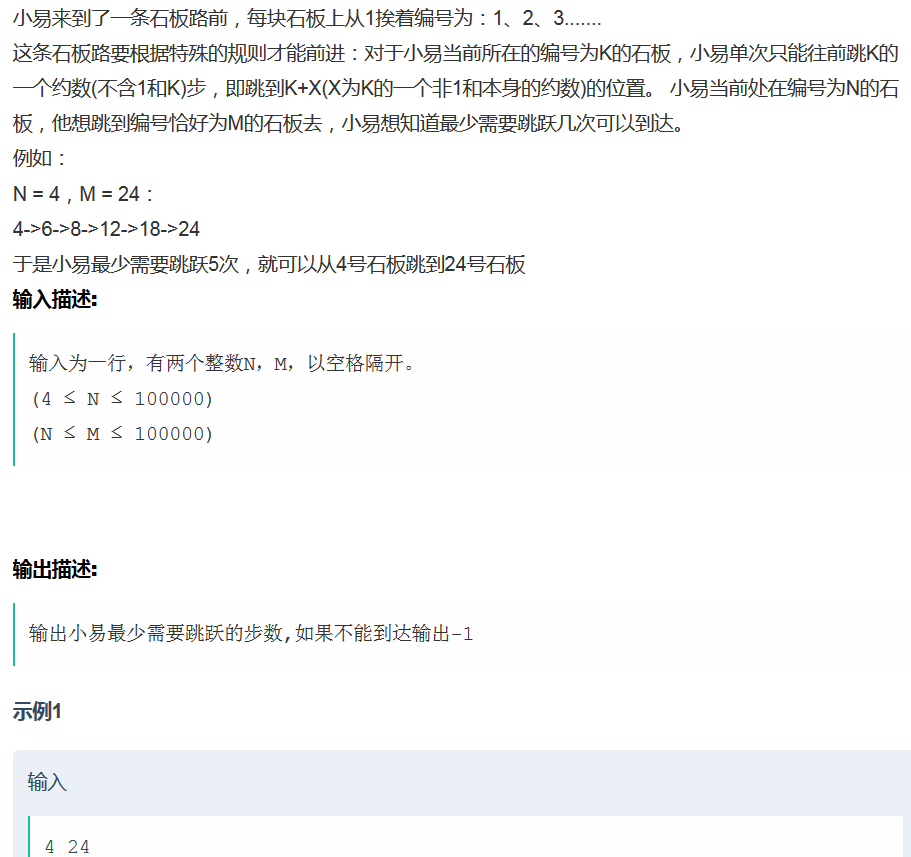思路：https://www.nowcoder.com/questionTerminal/4284c8f466814870bae7799a07d49ec8

代码：

import java.util.Scanner;

public class Solution {

public static void main(String[] args) {

Scanner sc = new Scanner(System.in);
while(sc.hasNext()){
int N = sc.nextInt();
int M = sc.nextInt();

int[] dp = new int;
for(int i=N; i<=M; i++){
dp[i] = Integer.MAX_VALUE;      //表示不可到达
}
dp[N] = 0;
for(int i=N; i<M; i++){
if(dp[i]==Integer.MAX_VALUE){
continue;
}
//计算约数
for(int j=2; j*j<=i; j++){
if(i%j==0){
if((i+j)<=M){
dp[i+j] = Math.min(dp[i+j], dp[i]+1);
}

if((i+i/j)<=M){
dp[i+i/j] = Math.min(dp[i+i/j], dp[i]+1);
}
}
}

}

int result = -1;
if(dp[M]!=Integer.MAX_VALUE){
result = dp[M];
}

System.out.println(result);

}

}

}

3、直方图包含的最大面积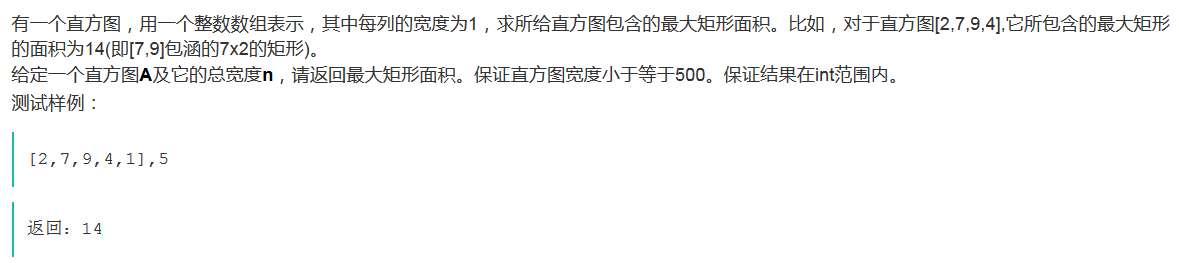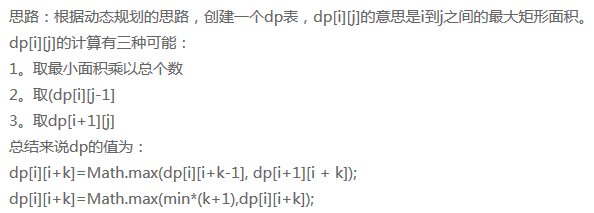（参考: http://blog.csdn.net/li563868273/article/details/51121169）

代码：

public int countArea(int[] A, int n) {

int[][] dp = new int[n][n];
//初始值
for(int i=0; i<n; i++){
dp[i][i] = A[i];
}

for(int k=1; k<n; k++){
for(int i=0; (i+k)<n; i++){

//得到该区间的最小值
int min = A[i];
for(int j=i; j<=(i+k); j++){
min = ((A[j]<min)?A[j]:min);
}

//比较3种情况，得到最大值
dp[i][i+k] = Math.max(dp[i+1][i+k], dp[i][i+k-1]);
dp[i][i+k] = Math.max(min*(k+1), dp[i][i+k]);
}
}

return dp[n-1];

}

4、路径问题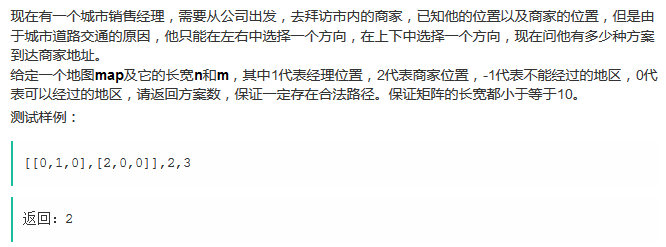思路：（参考“必备知识”中的二维DP问题）

代码：

public int countPath(int[][] map, int n, int m) {

int startX = 0;
int startY = 0;
int endX = 0;
int endY = 0;

for(int i=0; i<n; i++){
for(int j=0; j<m; j++){
if(map[i][j] == 1){
startX = j;
startY = i;
}else if(map[i][j] == 2){
endX = j;
endY = i;
}
}
}
//确定方向
int dirX = (startX<endX ? 1 : -1);
int dirY = (startY<endY ? 1 : -1);

int[][] count = new int[n][m];
count[startY][startX] = 1;

//确定竖边界的初始值
for(int i=startY+dirY; i!=(endY+dirY); i+=dirY){
if(map[i][startX]==-1){
count[i][startX] = 0;
}else{
count[i][startX] = count[i-dirY][startX];
}
}
//确定横边界的初始值
for(int j=startX+dirX; j!=(endX+dirX); j+=dirX){
if(map[startY][j]==-1){
count[startY][j] = -1;
}else{
count[startY][j] = count[startY][j-dirX];
}
}

for(int i=startY+dirY; i!=(endY+dirY); i+=dirY){
for(int j=startX+dirX; j!=(endX+dirX); j+=dirX){

if(map[i][j]==-1){
count[i][j] = 0;
}else{
count[i][j] = count[i-dirY][j] + count[i][j-dirX];
}

}
}

return count[endY][endX];

}

5、合唱团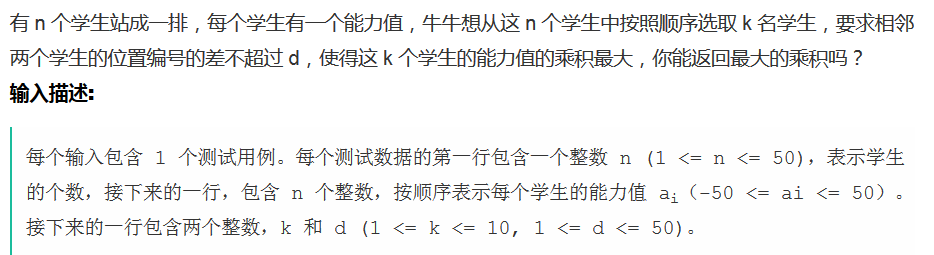解题思路： http://blog.csdn.net/fcxxzux/article/details/52138964


import java.util.Scanner;

public class Main {

public static void main(String[] args) {

Scanner sc = new Scanner(System.in);
while(sc.hasNext()){
int n = sc.nextInt();
int[] a = new int[n+1];
for(int i=1; i<=n; i++){
a[i] = sc.nextInt();
}
int k = sc.nextInt();
int d = sc.nextInt();

//dpMax[i][j]表示以第i个人为最后一个，已经选择了j个人了
long[][] dpMax = new long[n+1][k+1];
long[][] dpMin = new long[n+1][k+1];

long result = 0;
for(int i=1; i<=n; i++){
dpMax[i] = dpMin[i] = a[i];
for(int j=2; j<=k; j++){

if(i<j){
dpMax[i][j] = dpMin[i][j] = 0;
}else{
for(int m=i-1; m>=Math.max(i-d, 1); m--){
dpMax[i][j] = Math.max(dpMax[i][j], Math.max(dpMax[m][j-1]*a[i], dpMin[m][j-1]*a[i]));
dpMin[i][j] = Math.min(dpMin[i][j], Math.min(dpMax[m][j-1]*a[i], dpMin[m][j-1]*a[i]));
}
}

}

result = Math.max(result, dpMax[i][k]);
}

System.out.println(result);
}
}

}

6、剪气球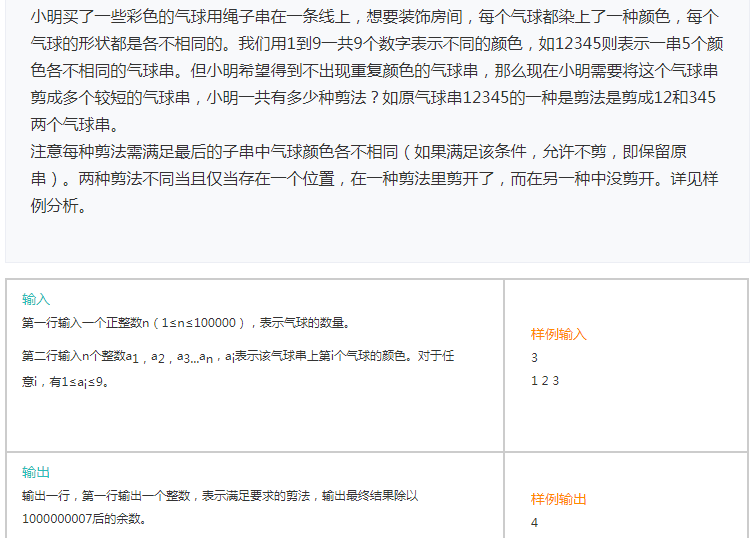题目来源：http://exercise.acmcoder.com/quesexcuse?paperId=213

思路：在计算dp[i+1]时，我们需要考虑第i+1个数可以和前面哪些数分到一起组成连续的子数组。（参考：http://blog.csdn.net/jacky_chenjp/article/details/63684427）

代码：

import java.util.Scanner;

public class Main {

public static void main(String[] args) {

Scanner sc = new Scanner(System.in);
while(sc.hasNext()){
int n = sc.nextInt();
int[] a = new int[n];
for(int i=0; i<n; i++){
a[i] = sc.nextInt();
}

int[] dp = new int[n+1];
dp = 1;
for(int i=1; i<=n; i++){
int[] count = new int;
for(int j=i-1; j>=0; j--){
count[a[j]]++;
if(count[a[j]]>1){
break;
}else{
dp[i] = (dp[i]+dp[j])%1000000007;
}
}

}

System.out.println(dp[n]);
}

}

}

7、最长公共子括号序列（来自: 牛客网）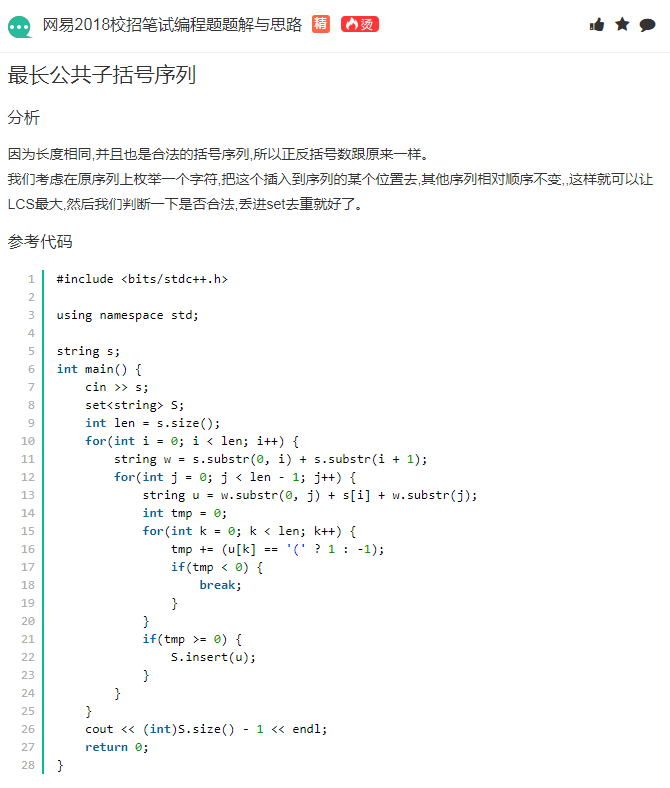展开全文• ## 动态规划算法

千次阅读 2015-08-02 09:45:57
动态规划过程是：每次决策依赖于当前状态，又随即引起状态的转移。一个决策序列就是在变化的状态中产生出来的，所以，这种多阶段最优化决策解决问题的过程就称为动态规划。 二、基本思想与策略 基本思想与分治法...
• 首先,本博客为原创作品,欢迎指导,随意转载,如果可以请转载时说明出处,附上...动态规划算法的基本思想与分治法类似，也是将待求解的问题分解为若干个子问题（阶段），按顺序求解子阶段，前一子问题的解，为后一子问题...
• ## 夜深人静写算法（二）- 动态规划

万次阅读 多人点赞 2017-12-28 14:57:36DP
• ## 剑指Offer——动态规划算法

万次阅读 多人点赞 2016-08-03 15:24:27
剑指Offer——动态规划算法什么是动态规划？ 和分治法一样，动态规划（dynamicprogramming）是通过组合子问题而解决整个问题的解。 分治法是将问题划分成一些独立的子问题，递归地求解各子问题，然后合并子问题的解...
• ## 动态规划算法详解

千次阅读 2016-07-17 17:12:38
动态规划的介绍 动态规划一般也只能应用于有最优子结构的问题。最优子结构的意思是局部最优解能决定全局最优解(对有些问题这个要求并不能完全满足，故有时需要引入一定的近似)。简单地说，问题能够分解成子问题来...
• 动态规划算法是通过拆分问题，定义问题状态和状态之间的关系，使得问题能够以递推（或者说分治）的方式去解决。 什么是动态规划 动态规划（Dynamic Programming）对于子问题重叠的情况特别有效，因为它将子问题...
• 动态规划过程是：每次决策依赖于当前状态，又随即引起状态的转移。一个决策序列就是在变化的状态中产生出来的，所以，这种多阶段最优化决策解决问题的过程就称为动态规划。 二、基本思想与策略 基本思想与分治法...
• 动态规划算法 定义 动态规划（Dynamic programming）是一种在数学、计算机科学和经济学中使用的，通过把原问题分解为相对简单的子问题的方式求解复杂问题的方法。 动态规划常常适用于有重叠子问题和最优子结构...
• 动态规划算法一、基本概念 动态规划过程是：每次决策依赖于当前状态，又随即引起状态的转移。一个决策序列就是在变化的状态中产生出来的，所以，这种多阶段最优化决策解决问题的过程就称为动态规划。二、基本思想与...
• 0-1 背包问题：给定 n 种物品和一个容量为 C 的背包，物品 i 的重量是 wi，其价值为 vi 。 问：应该如何选择装入背包的物品，使得装入背包中的物品的总价值最大？01背包
• ## 初级算法-动态规划

千次阅读 2018-07-15 17:48:43
本篇主要介绍动态规划解题思路 概括 动态规划主要是解一些递归问题，也就是将递归写成非递归方式，因为编辑器无法正确对待递归，递归方法会导致很多计算结果被重复计算，比如菲波那切数列。 所以动态规划的解题...leetcode 打家劫舍...